International
Tables for
Crystallography
Volume A
Space-group symmetry
Edited by M. I. Aroyo

International Tables for Crystallography (2015). Vol. A, ch. 1.4, pp. 50-51

## Section 1.4.2.1. Symbols for symmetry operations

M. I. Aroyo,a G. Chapuis,b B. Souvignierd and A. M. Glazerc

#### 1.4.2.1. Symbols for symmetry operations

| top | pdf |

Given the analytical description of the symmetry operations by matrix–column pairs, their geometric meaning can be determined following the procedure discussed in Section 1.2.2. The notation scheme of the symmetry operations applied in the space-group tables was designed by W. Fischer and E. Koch, and the following description of the symbols partly reproduces the explanations by the authors given in Section 11.1.2of IT A5. Further explanations of the symbolism and examples are presented in Section 2.1.3.9.

The symbol of a symmetry operation indicates the type of the operation, its screw or glide component (if relevant) and the location of the corresponding geometric element (cf. Section 1.2.3and Table 1.2.3.1for a discussion of geometric elements). The symbols of the symmetry operations explained below are based on the Hermann–Mauguin symbols (cf. Section 1.4.1.4), modified and supplemented where necessary.

 The symbol for the identity mapping is 1. A translation is symbolized by the letter t followed by the components of the translation vector between parentheses. Example: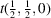represents a translation by a vector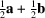, i.e. a C centring. A rotation is symbolized by a number n = 2, 3, 4 or 6 (according to the rotation angle 360°/n) and a superscript + or −, which specifies the sense of rotation (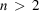). The symbol of rotation is followed by the location of the rotation axis. Example: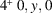indicates a rotation of 90° about the line 0, y, 0 that brings point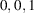onto point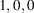, i.e. a counter-clockwise rotation (or rotation in the mathematically positive sense) if viewed from point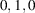to point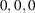. A screw rotation is symbolized in the same way as a pure rotation, but with the screw part added between parentheses. Example: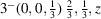indicates a clockwise rotation of 120° around the line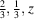(or rotation in the mathematically negative sense) if viewed from the point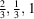towards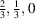, combined with a translation of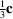. A reflection is symbolized by the letter m, followed by the location of the mirror plane. A glide reflection in general is symbolized by the letter g, with the glide part given between parentheses, followed by the location of the glide plane. These specifications characterize every glide reflection uniquely. Exceptions are the traditional symbols a, b, c, n and d that are used instead of g. In the case of a glide plane a, b or c, the explicit statement of the glide vector is omitted if it is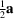,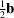or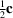, respectively. Examples: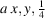means a glide reflection with glide vectorand through a plane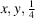;denotes a glide reflection with glide part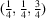and the glide plane d at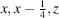. An inversion is symbolized byfollowed by the location of the inversion centre. A rotoinversion is symbolized, in analogy with a rotation, by,orand the superscript + or −, again followed by the location of the (rotoinversion) axis. Note that angle and sense of rotation refer to the pure rotation and not to the combination of rotation and inversion. In addition, the location of the inversion point is given by the appropriate coordinate triplet after a semicolon. Example: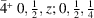means a 90° rotoinversion with axis at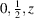and inversion point at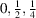. The rotation is performed in the mathematically positive sense when viewed from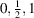towards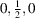. Therefore, the rotoinversion maps pointonto point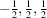.

The notation scheme is extensively applied in the symmetry-operations blocks of the space-group descriptions in the tables of Chapter 2.3. The numbering of the entries of the symmetry-operations block corresponds to that of the coordinate triplets of the general position, and in space groups with primitive cells the two lists contain the same number of entries. As an example consider the symmetry-operations block of the space group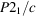shown in Fig. 1.4.2.1. The four entries correspond to the four coordinate triplets of the general-position block of the group and provide the geometric description of the symmetry operations chosen as coset representatives ofwith respect to its translation subgroup.Figure 1.4.2.1 | top | pdf |General-position and symmetry-operations blocks for the space group, No. 14 (unique axis b, cell choice 1). The coordinate triplets of the general position, numbered from (1) to (4), correspond to the four coset representatives of the decomposition ofwith respect to its translation subgroup, cf. Table 1.4.2.6. The entries of the symmetry-operations block numbered from (1) to (4) describe geometrically the symmetry operations represented by the four coordinate triplets of the general-position block.

For space groups with conventional centred cells, there are several (2, 3 or 4) blocks of symmetry operations: one block for each of the translations listed below the subheading Coordinates'. Consider, for example, the four symmetry-operations blocks of the space group Fmm2 (42) reproduced in Fig. 1.4.2.2. They correspond to the four sets of coordinate triplets of the general position obtained by the translations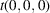,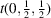,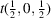and, cf. Fig. 1.4.2.2. The numbering scheme of the entries in the different symmetry-operations blocks follows that of the general position. For example, the geometric description of entry (4) in the symmetry-operations block under the heading For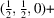set' of Fmm2 corresponds to the coordinate triplet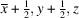, which is obtained by addingto the translation part of the printed coordinate triplet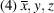(cf. Fig. 1.4.2.2).Figure 1.4.2.2 | top | pdf |General-position and symmetry-operations blocks as given in the space-group tables for space group Fmm2 (42). The numbering scheme of the entries in the different symmetry-operations blocks follows that of the general position.